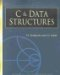# Strings

## STRINGS AS AN ARRAY OF CHARACTERS

### Introduction

A string is defined as an array of characters. Strings are terminated by the special character ‘o’; this is called a null parameter or null terminator. When you declare the string, you should ensure that you should have sufficient room for the null terminator. The null terminator has ASCII value 0.

Program

```main ( )
{
char s1; \ A
char s2;
char ch;
int cnt = 0;
s1 = "Hello"; \ B
printf ("%s
", s1); \ C
s2 = {'H', 'e', 'l', 'l', 'o'} \ D
printf("%s
", s2); \ E
while ( (ch = getchar() )! = '#' && (cnt < 6-1) ) \ F
s1[cnt++] = ch; \ G
s1[cnt] = ''; \ H
}
```

Explanation

1. The size of the string is 6, which is the last element terminator, so you can use only 5 positions.
2. In statement B, the string "Hello" is assigned so that the array elements are

```H e l l o
```
3. The null terminator is appended automatically.
4. Statement B puts the data in a string using standard array notation.
5. You can print a string using the placeholder %s; the string is printed until it encounters a null character.
6. The while loop in statement H inputs the string by reading character by character.
7. The function getchar returns the character.
8. Note that the counter is incremented up to 5 so as to accommodate the last null terminator.
9. The null terminator is put in place by statement H.
10. The while loop can be terminated before counter 5 by putting in the # character.

Points to Remember

1. A string is a character array with a null terminator at the end.
2. You can initialize the array using different methods.

## STRING DEFINITION

### Introduction

A string can be defined using a character array or a pointer to characters. Although the two definitions look similar, they are actually different.

Program

```main ( )
{
char * s1 = "abcd"; \ A
char s2[] = "efgh"; \ B
printf( "%s %16lu
, s1, s1); \ C
printf( "%s %16lu
, s2, s2); \ D
s1 = s2; \ E
printf( "%s %16lu
, s1, s1); \ F
printf( "%s %16lu
, s2, s2); \ G
}
```

Explanation

1. Statement A declares s1 as a pointer to a character. When this definition is encountered, the compiler allocates space for the string abcd; the base address of the string is assigned to s1, which is the pointer variable.
2. Statement B declares s2 as a character array. The size of the array is 5 because of an additional null terminator in this case. Also, a space of 5 characters is allocated and the base address is given to s2, which is the pointer constant. During the lifetime of the program, we cannot change the value of s2.
3. The allocation for s1 is the allocation required by the pointer variable.
4. Statement C prints s1, using two place holders: %s and %16lu. Using %s, you will print the string as "abcd". Using %16lu you will print the base address of the string.
5. Statement E assigns a base address of s2 to s1; that is possible because s1 is a variable.

Point to Remember

When the string is declared as a character pointer, a space is allocated for the pointer variable, which holds the base address of the string.

## STRINGS AS PARAMETERS

### Introduction

The string can be passed to a function just as in a normal array. The following examples are used for printing the number of characters in the string:

Program

```main ( )
{
char s1 = "abcde ";
int cnt = 0;
cnt = cnt_str(s1); \ A
printf( " total characters are %d
", cnt);
}
int cnt_str(char s1[]); \ B
{
int cn = 0;
while ( (cn < 6) && s1[cn]! = '')
cn++;
return(cn);
}
```

Explanation

1. A function, cnt_str, calculates the number of characters in a string. The string is passed just as a character array. When the array is passed, the base address of the array is actually what gets passed.
2. Statement B is called to a function in which s1 is passed just as a normal array.C & Data Structures (Charles River Media Computer Engineering)
ISBN: 1584503386
EAN: 2147483647
Year: 2006
Pages: 232